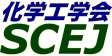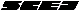## \$B9V1i%W%m%0%i%`!J%;%C%7%g%sJL!K(B

### \$B%7%s%]%8%&%`(B \$B!c?7\$?\$JE83+\$r7^\$(\$k%_%-%7%s%05;=Q\$NM}O@!&B,Dj!&AuCV!d(B

#### S201-S219

 \$B%*!<%,%J%\$%6!<(B \$B2CF#Dw?M(B(\$BL>9)Bg(B)\$B!\$:#Cf>HM:(B(\$B?@9]4D6-%=%j%e!<%7%g%s(B)

\$B6aG/%_%-%7%s%05;=Q\$K4X\$7\$F!"?7\$?\$J;kE@\$+\$i\$NM}O@!"3FMh@=IJ\$NIJR2p\$@\$1\$G\$J\$/?<\$\$5DO@\$b4|BT\$7\$^\$9!#\$^\$?!"%S%G%*\$r4^\$`%W%l%<%s%F!<%7%g%s\$r4|BT\$7\$^\$9!#F02h>^\$NBP>]\$O!"N3;R!&N.BN%W%m%;%9It2q\$N%7%s%]%8%&%`\$N\$I\$l\$+\$K\$F>R2p\$5\$l\$?%S%G%*:nIJ\$N\$&\$A!"F02h>^\$K1~Jg\$5\$l\$?\$b\$N\$rBP>]\$H\$7\$^\$9\$N\$G!"@Q6KE*\$K1~Jg\$7\$F\$\$\$?\$@\$1\$l\$P9,\$\$\$G\$9!#FC\$K650iMQ\$N%S%G%*:nIJ\$OIt2q2#CG\$N%7%s%]%8%&%`!VN3;R!&N.BN%W%m%;%98&5f!&650i\$N?7\$7\$\$2]Bj\$HJ}8~!W\$K\$4H/I=\$/\$@\$5\$\$!#(B

\$B:G=*99?7F|;~!'(B2010-09-03 10:20:57
\$B9V1i(B
\$B;~9o(B
\$B9V1i(B
\$BHV9f(B
\$B9V1iBjL\!?H/I=\$B%-!<%o!<%I(B\$BJ,N`(B
\$BHV9f(B
\$B\$BHV9f(B
S\$B2q>l(B \$BBh(B2\$BF|(B
(9:00\$B!A(B10:00)\$B!!(B(\$B:BD9(B \$B2CF#Dw?M(B)
9:00\$B!A(B 9:20S201\$B@55U8r8_2sE>YxYBMc\$N:.9gFC@-(B
(\$B?@8MBg1!(B) \$B!{(B(\$B@5(B)\$B8VED(B \$B1YG7(B \$B!&(B (\$B%"!<%k%U%m!<(B) (\$B@5(B)\$BC]ED(B \$B9((B \$B!&(B (\$B:eBg(B) (\$B@5(B)\$BJ?ED(B \$BM:;V(B \$B!&(B (\$B?@8MBg1!(B) (\$B@5(B)\$BNkLZ(B \$BMN(B
angular reciprocation
chaotic mixing
CFD
S-411029
9:20\$B!A(B 9:40S202\$BBg7?Mc\$rMQ\$\$\$?JP?43IYB\$K\$*\$1\$kF0NO\$*\$h\$S:.9gFC@-\$H%9%m%C%7%s%08=>](B
(\$B2#9qBg1!9)(B) \$B!{(B(\$B@5(B)\$B?N;V(B \$BOBI'(B \$B!&(B (\$B3X(B)\$B1vC+(B \$BD> \$B!&(B \$BEDCf(B \$BM*B@(B \$B!&(B (\$B@5(B)\$B;03Q(B \$BN4B@(B \$B!&(B (\$B@5(B)\$B>e%N;3(B \$B<~(B
eccentric mixing
large type impeller
power consumption
S-41192
9:40\$B!A(B 10:00S203\$BN.BN:.9g35G0\$N0lHL2=(B
(\$B:eBg1!4p9)(B) \$B!{(B(\$B3X(B)\$B2,ED(B \$BJ8B@O/(B \$B!&(B (\$BEl%l(B) \$B@P4](B \$BBs(B \$B!&(B (\$B:eBg1!4p9)(B) (\$B@5(B)\$B66K\(B \$B=SJe(B \$B!&(B (\$B@5(B)\$B0f>e(B \$B5AO/(B
fluid mixing
periodic reaction
concentration pattern
S-41287
(10:00\$B!A(B11:00)\$B!!(B(\$B:BD9(B \$B66K\=SJe(B)
10:00\$B!A(B 10:20S204\$B:81&HsBP>N\$N8rBX79
(\$BL>9)Bg(B) (\$B@5(B)\$B2CF#(B \$BDw?M(B \$B!&(B \$B!{(B(\$B3X(B)\$B2CF#(B \$B5*9,(B \$B!&(B (\$B@5(B)\$BB?ED(B \$BK-(B \$B!&(B (\$B@5(B)\$BD9DE(B \$BM:0lO:(B
mixing
agitation
turbulent
S-4113
10:20\$B!A(B 10:40S205\$BMpN.>uBV\$K\$*\$1\$k=jMWF0NO\$HAm3g:.9g@-G=;XI8!&:.9g%9%Z%/%H%k;XI8\$N4X78(B
(\$BEl9)Bg1!M}9)(B) \$B!{(B(\$B3X(B)\$BJR6M(B \$B>-C#(B \$B!&(B (\$B@5(B)\$BBg@n86(B \$B??0l(B \$B!&(B (\$B@5(B)\$B5H@n(B \$B;KO:(B \$B!&(B (\$B@5(B)\$B>.@n(B \$B9@J?(B
Mixing
Power consumption
Mixing Performance
S-41775
10:40\$B!A(B 11:00S206\$BFsCJMc\$K\$*\$1\$k
(\$BL>9)Bg(B) (\$B@5(B)\$B2CF#(B \$BDw?M(B \$B!&(B \$B!{(B(\$B3X(B)\$B>.H*(B \$B\$"\$:\$5(B \$B!&(B (\$B@5(B)\$BB?ED(B \$BK-(B \$B!&(B (\$B@5(B)\$BD9DE(B \$BM:0lO:(B
mixing
dual impeller
laminar
S-4146
(11:00\$B!A(B12:00)\$B!!(B(\$B:BD9(B \$B9b669,;J(B)
11:00\$B!A(B 11:20S207\$B9ZJl\$K\$h\$k%(%?%N!<%kH/9Z\$K5Z\$\\$93IYBQrCG8z2L(B
(\$B?@8MBg1!9)(B) \$B!{(B(\$B3X(B)\$BFbED(B \$BFX;R(B \$B!&(B (\$B3X(B)\$B0KF#(B \$BM'65(B \$B!&(B (\$B3X(B)\$BKYED(B \$B=_;K(B \$B!&(B (\$B@5(B)\$BKY9>(B \$B9';K(B \$B!&(B (\$B@5(B)\$B2.Ln(B \$B@i=)(B \$B!&(B (\$B@5(B)\$BBgB<(B \$BD>?M(B
Bioethanol
Shear stress
Fermentation
S-41548
11:20\$B!A(B 11:40S208Analysis of Enhancement Mechanism on Mixing with Baffles by use of Three-Dimensional Streak Visualization
(\$B:eBg1!4p9)(B) \$B!{(B(\$B3X(B)Onyango Oketch \$B!&(B (\$B@5(B)\$B66K\(B \$B=SJe(B \$B!&(B (\$B@5(B)\$B0f>e(B \$B5AO/(B
Fluid Mixing
Streak Visualization
Baffle
S-41467
11:40\$B!A(B 12:00S209\$B%?!<%S%sMc\$rMQ\$\$\$?3IYBAeFb\$N(Bshear-thinning\$B@-N.BNCfHyN3;RJ,;6FC@-(B
(\$B?@8MBg1!9)(B) \$B!{(B(\$B3X(B)\$B:eLn(B \$B?-Bg(B \$B!&(B (\$BBgF|K\0u:~(B) (\$B@5(B)\$BAj_7(B \$B1I \$B!&(B (\$B?@8MBg1!9)(B) (\$B@5(B)\$BKY9>(B \$B9';K(B \$B!&(B (\$B@5(B)\$B7'C+(B \$B595W(B \$B!&(B (\$B@5(B)\$BBgB<(B \$BD>?M(B
mixing
non newton(shear-thinning)fluid
dispersion
S-41856

(13:00\$B!A(B13:40)\$B!!(B(\$B;J2q(B \$B:#Cf>HM:(B)
13:00\$B!A(B 13:40S213[\$BE8K>9V1i(B] \$B9bJ,;R:`NA\$NMOM;:.N}(B:\$B8=>u\$H:#8e\$NE8K>(B
(\$B6eBg(B) \$B!{(B(\$B@5(B)\$B3a86(B \$BL->0(B
Melt Mixing
Mixing Theory
Polymer Materials
S-4173
(13:40\$B!A(B14:40)\$B!!(B(\$B:BD9(B \$B?N;VOBI'(B)
13:40\$B!A(B 14:00S215\$B%O%\$%V%j%C%IMc=E9g4o\$K\$*\$1\$k>xH/LL@Q\$*\$h\$SI=LL99?7B.EY\$N8!F\$(B
(\$BF|N)(B) \$B!{(B(\$B@5(B)\$B2,(B \$B7{0lO:(B \$B!&(B (\$B@5(B)\$B>>Hx(B \$B=SL@(B \$B!&(B (\$B@5(B)\$B>e@n(B \$B>-9T(B \$B!&(B (\$BF|N)%W%i%s%H%F%/%N%m%8!<(B) \$B0KF#(B \$BGnG7(B
CFD
Free Surface
Polymerization
S-41112
14:00\$B!A(B 14:20S216\$B%N%s%(%l%a%s%H%_%-%5!<\$K\$*\$1\$k:.9gFC@-\$K\$D\$\$\$F\$N;0
(\$B7DXfBgM}9)(B) \$B!{(B(\$B3X(B)\$B1|ED(B \$BFA9,(B \$B!&(B (\$B3X(B)\$B5WJ](B \$B5.B'(B \$B!&(B (\$B@5(B)\$BF#2,(B \$B:;ET;R(B \$B!&(B (\$B@5(B)\$B2#?9(B \$B9d(B \$B!&(B (\$B@5(B)\$B?"ED(B \$BMx5W(B
3D visualization
non-element mixer
chaotic mixing
S-41386
14:20\$B!A(B 14:40S217\$BDL5\$3IYB\$K\$*\$1\$kJ?6Q5\$K"7B\$K5Z\$\\$9A`:n>r7o\$N1F6A(B
(\$B;37ABg1!(B) \$B!{(B(\$B3X(B)\$BC+K\(B \$B;j(B \$B!&(B (\$B@5(B)\$B9b66(B \$B9,;J(B \$B!&(B (DIC) \$B4X:,(B \$B6Q(B
Gas Dispersion
Diameter of Bubble
Gas Flow Rate
S-41751
(14:40\$B!A(B15:20)\$B!!(B(\$B:BD9(B \$B8VED1YG7(B)
14:40\$B!A(B 15:00S218LDV\$B\$*\$h\$S(BPIV\$B\$rMQ\$\$\$?2sE>1_E{7?1U1UCj=PAuCV\$K\$*\$1\$kN.F0>uBV\$N7WB,(B
(\$B2#9qBg1!9)(B) \$B!{(B(\$B3X(B)\$BT"0f(B \$B2BF`;R(B \$B!&(B (\$B@5(B)\$B;03Q(B \$BN4B@(B \$B!&(B (\$B@5(B)\$B?N;V(B \$BOBI'(B \$B!&(B (\$B@5(B)\$B>e%N;3(B \$B<~(B \$B!&(B (\$B86;RNO5!9=(B) \$B2C@%(B \$B7r(B \$B!&(B \$BC]Fb(B \$B@59T(B \$B!&(B \$B:4Ln(B \$BM:0l(B
mixing
liquid-liquid extraction
fluid behavior
S-41191
15:00\$B!A(B 15:20S219CFD\$B\$rMQ\$\$\$?O"B3<0%K<4%(%/%9%H%k!<%@\$N:.N}@-G=I>2A(B
(\$B%i%\$%*%s(B) \$B!{(B(\$B@5(B)\$B1JLn(B \$BC#@8(B \$B!&(B (\$B;37ABg9)(B) (\$B@5(B)\$B9b66(B \$B9,;J(B \$B!&(B (\$B@5(B)\$B>>ED(B \$B7=8g(B \$B!&(B (\$B%"!<%k%U%m!<(B) (\$B@5(B)\$BC]ED(B \$B9((B(C) 2010 (\$B
E-mail: inquiry-42fwww3.scej.org
This page was generated byeasp 2.28; proghtml 2.28 (C)1999-2008 kawase